# Catch up on the latest AI articles# I Want To Use GAN More Easily.

3 main points
✔️ Create a generative model from a sketch
✔️ Achieved high accuracy compared to baseline
✔️ Expected to be improved in the future

written by Sheng-Yu WangDavid BauJun-Yan Zhu
(Submitted on 5 Aug 2021)

Subjects: Computer Vision and Pattern Recognition (cs.CV); Machine Learning (cs.LG)code：The images used in this article are from the paper or created based on it.

## first of all

GANs have developed in technology and can produce high-quality images. Of course, there are some limitations, but the usefulness of GAN has been increasing. However, with some limitations, the question arises as to whether it can be used by ordinary users. It may seem like a big barrier to use such a highly useful method without a specialist. For example, how can a user who creates work with cats generate special cat images with specific poses, such as a lying cat, a left-facing cat, etc.? To obtain such customized generation, this paper takes up this challenge by proposing the task of creating a generative model from a hand-drawn sketch. Instead of creating a single image from a sketch, we ask whether it is possible to create a generative model of a realistic image from a hand-drawn sketch. As shown in the figure below, with just four hand-drawn sketches, we can change the pose of an object or zoom in on a cat's face.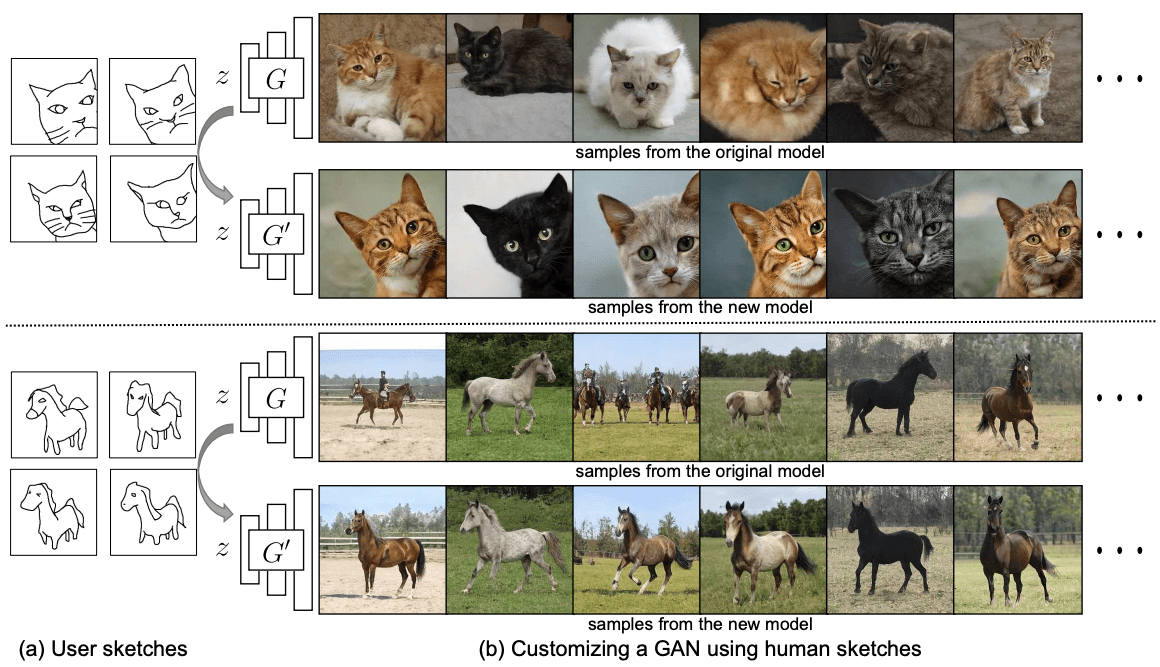Below is an overview image of this task.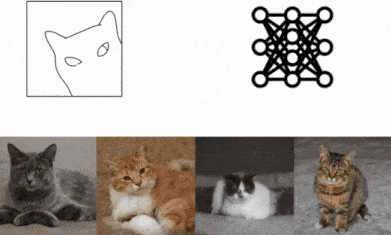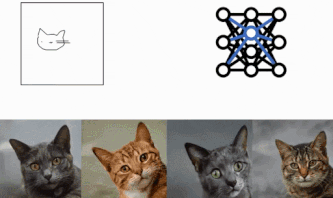## technique

We will be using three main components.

1. To create a GAN model from a sketch, we introduce a cross-domain adversarial loss using a domain transformation network, as before, since it is a mismatch between the training data (= sketch) and the output (= image). However, the simple use of this loss leads to the problem that the behavior of the model itself changes considerably and unrealistic results are obtained, so we propose an improved loss. →Cross-Domain Adversarial Learning
2. To preserve the content of the original dataset and its diversity, we train the model by applying image space regularization. →Image Space Regularization
3. To reduce model overfitting, updates are limited to specific layers and data augmentation is performed. →Optimization

The overall learning process is shown in the figure below.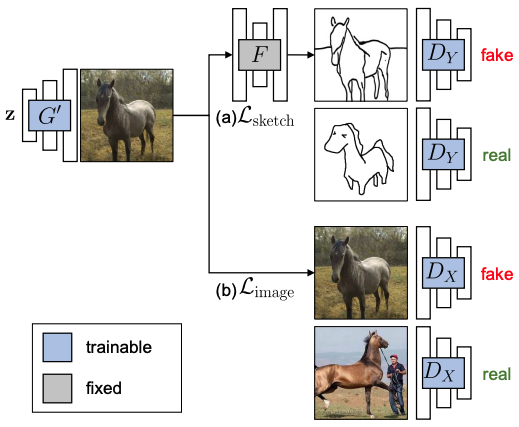Let X, Y be a domain consisting of images and sketches, respectively. We collect a large training image x~$p_(data)$(x) and a few human sketches y~$p_(data)$(y). Let $G(z;θ)$ be a pre-trained GAN that generates an image x from a low-dimensional code z. We want to create a new GAN model $G(z;θ')$ where the output image still follows the same data distribution of X, while the sketch version of the output image resembles the data distribution of Y. This network can be trained using input-output pairs such as a photograph and its sketch, but instead we will use a cross-domain image-to-sketch image transformation network F: X → Y (F: Photosketch). To bridge the gap between the sketch training data and the image generation model, we exploit cross-domain adversarial loss so that the generated image matches the sketch Y . Before passing to the discriminator, the output of the generator is transferred to the sketch by a pre-trained image-sketch network F (see below).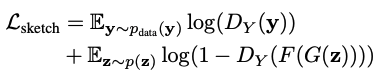### Image Space Regularization

Loss to sketch alone will only force the shape of the generated image to match the sketch. This means that the image quality and the diversity of the generation can be significantly reduced. To solve this problem, we add an adversarial loss that compares the output to a training set of the original model.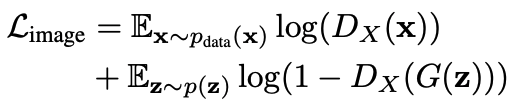where the discriminant $D_(X)$ is used to maintain the quality and diversity of the model output and to match the user's sketch.

We have also experimented with weight regularization, using the loss in the lower equation to explicitly penalize for large variations. We find that this does not actually improve accuracy, but causes performance degradation. However, our results suggest that applying either weight regularization or image space regularization is important for balancing image quality and shape matching.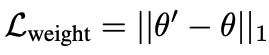### optimization

To prevent model overfitting and speed up fine-tuning, StyleGAN2 changed only the weights of the mapping network and remapped z ∼ N (0, I) into different intermediate potential spaces (W-space). Furthermore, they used a pre-trained Photosketch network F and fixed the weights of F through training. They experimented with a strategy of minimal augmentation on the training sketches and found that slight augmentation performed better in scene tests. Transformed enhancement was used in this study.

The final optimization equation is as follows

We set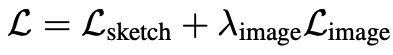$λ_(image)$ = 0.7 to control for the importance of the image-space regularization term. We train a new set of weights $G(z; θ')$ in the following minimax.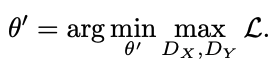## experiment

### data set

To enable large-scale quantitative evaluation, we build a dataset of model sketches with a distribution of correct answers defined as follows: images of LSUN horses, cats, and churches are converted into sketches using PhotoSketch, and as shown in the figure below, 30 sketches with similar shapes and poses are sets are hand-selected and used as user input. To define the target distribution, we further hand-selected 2,500 images that matched the input sketches. Only the specified 30 sketches are accessible, and the set of 2,500 real images is a distribution of targets that have never actually been seen.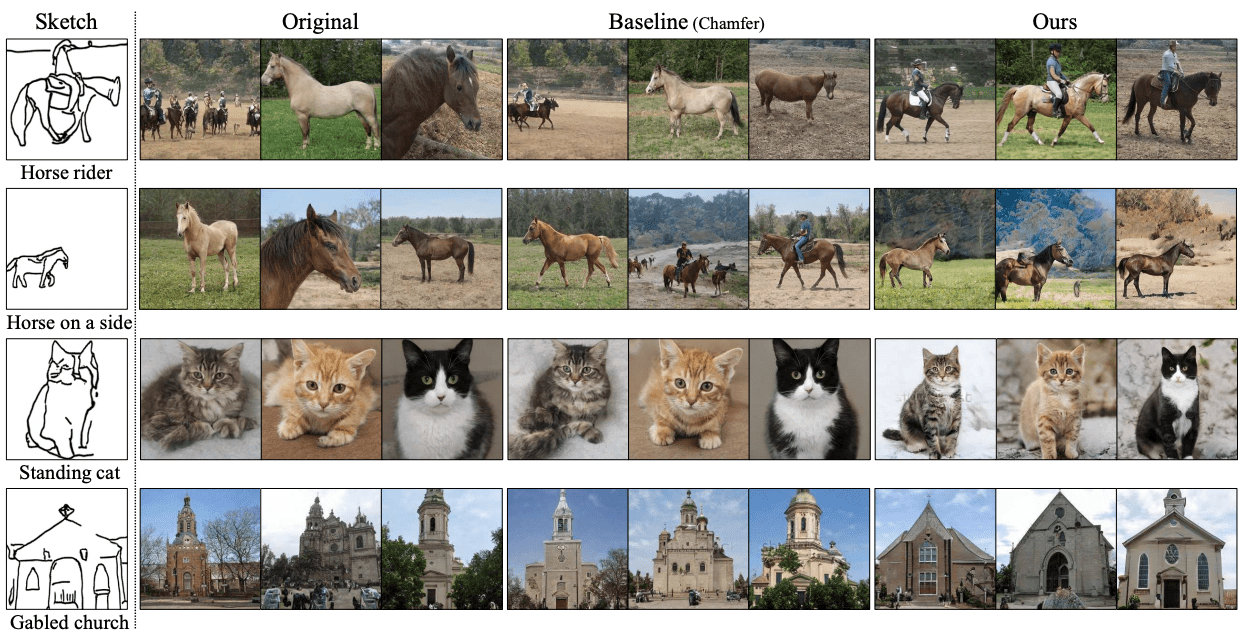### valuation index

We evaluate the model based on the Frechet Inception Distance (FID) between the generated images and the evaluation set, which measures the similarity of the distribution between the two sets and provides an indication of the diversity and quality of the generated images and how well the images match the sketch. FID is a measure of the similarity of the distributions between two sets of images.

### baseline

Similar to the vector arithmetic method proposed by Radford et al. we evaluate the effect of customizing the model output by shifting the potential $w_(new)$ = w + $∆w$ using a constant vector $∆w$ obtained by averaging samples similar to the user's sketch. ($L_(sketch)$+$L_(image)$ as Full (w/o aug.), $L_(sketch)$+$L_(image)$+aug. as Full (w/ aug.))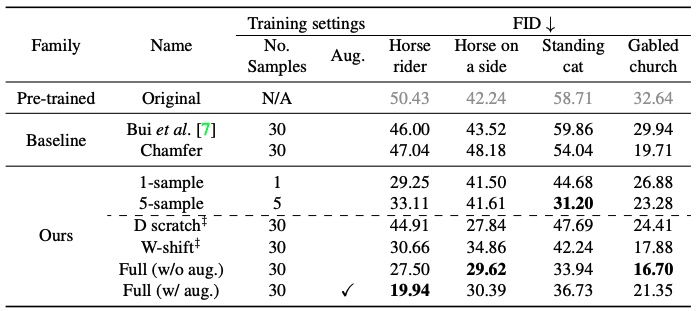The table shows the quantitative comparison. We can see that the results are consistent with the comparison shown in the figure above. We can see that the baseline methodology is not consistent with the user's sketch.

## Ablation Research

We study the effect of regularization methods and data augmentation. The results are shown in the table.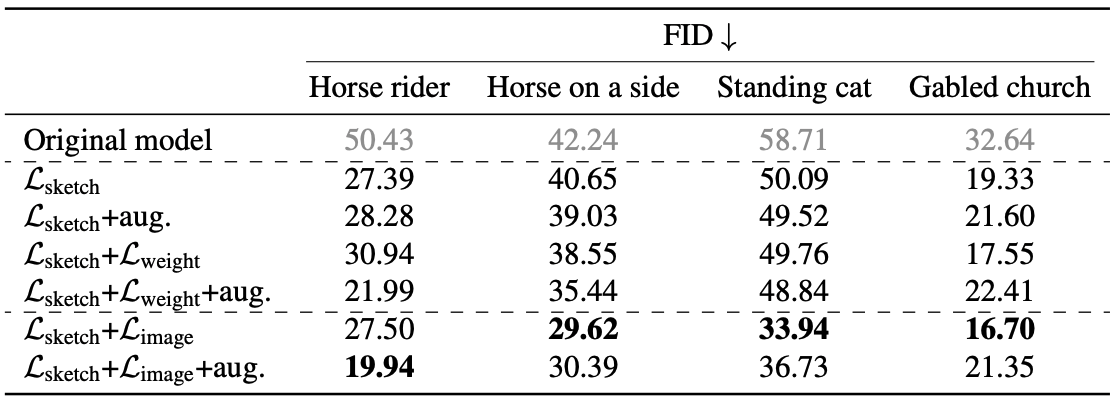For sketches generated from Photosketch, we see that augmentation does not necessarily improve performance. When applying image regularization, the model of a horse rider benefits from augmentation, but when generating models of a horse on its side, a standing cat, etc., the results are better without augmentation.

### Comparison of regularization methods

The regularization methods, $L_(image)$ or $L_(weight)$, improve FID over models trained with $L_(sketch)$ alone, but the models trained with image regularization are better than those trained with $L_(weight)$. This is consistent with the above, as the results for models trained with and without regularization are shown in the figure below.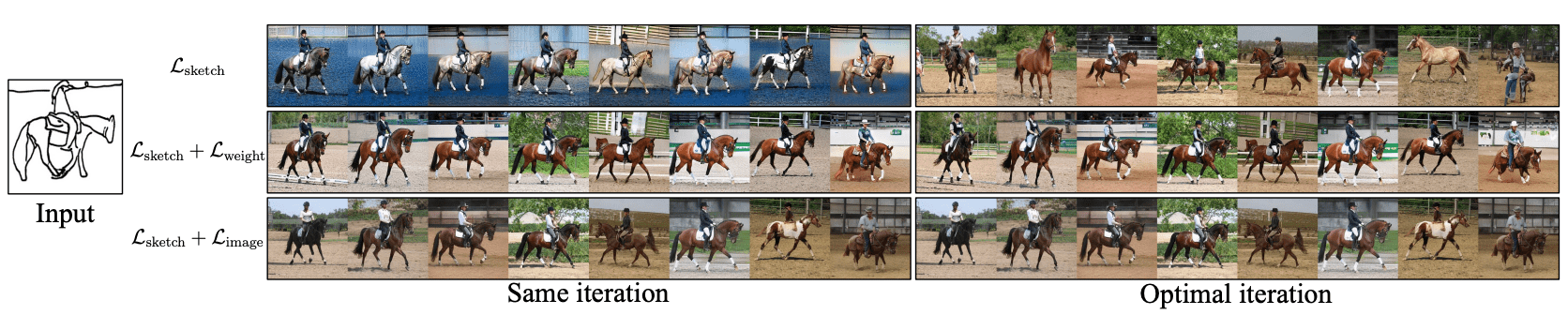## summary

We propose a method that allows users to create customized generative models using ready-made trained models and cross-domain training. In our method, a single handwritten sketch is used as an input, and even a novice user can create a generative model of that sketch.

However, this method is not able to work for all sketches. For example, if you test it on a Picasso sketch of a horse, it will fail. Picasso's sketch is not perfect, for example, because it is drawn in a unique style, which may be the reason for the failure. Also, while we have flexible control over the shape and pose, we have not been able to customize other characteristics such as color and texture. However, I think this area will be improved in the future.

If you have any suggestions for improvement of the content of the article,